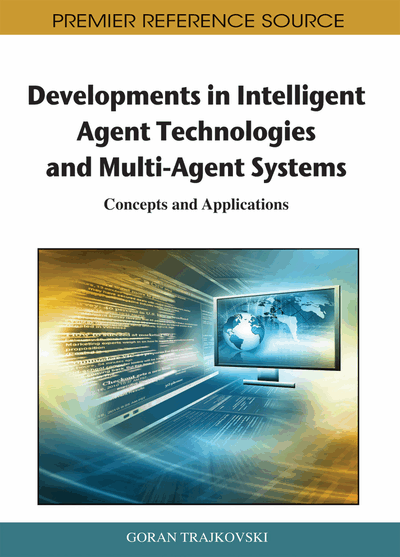# Quantifying Disorder in Networks: The von Neumann Entropy

Filippo Passerini (Humboldt-University, Germany) and Simone Severini (University College London, UK)
DOI: 10.4018/978-1-60960-171-3.ch005
Available
\$37.50
No Current Special Offers

## Abstract

The authors introduce a novel entropic notion with the purpose of quantifying disorder/uncertainty in networks. This is based on the Laplacian and it is exactly the von Neumann entropy of certain quantum mechanical states. It is remarkable that the von Neumann entropy depends on spectral properties and it can be computed efficiently. The analytical results described here and the numerical computations lead us to conclude that the von Neumann entropy increases under edge addition, increases with the regularity properties of the network and with the number of its connected components. The notion opens the perspective of a wide interface between quantum information theory and the study of complex networks at the statistical level.
Chapter Preview
Top

## Introduction

The von Neumann entropy (or equivalently quantum entropy) was defined by von Neumann (1955) in his fundational work in quantum mechanics. Nowadays the von Neumann entropy is an important tool in quantum information theory (see Nielsen and Chuang (2001); Ohya and Petz (1993)). In the present work we first associate a graph to a quantum state, injectively. Then, we study the von Neumann entropy of the state itself. Since the information contained in the state is nothing more but the information contained in the graph, with an easy abuse of language, we can say that we study the von Neumann entropy of the graph.

Our purpose is to get a feeling about the properties of a network that are readable through the Neumann entropy. During our discussion, we will guess that the properties highlighted by the entropy are related, even if not in a clear way, to the amount of symmetry in a graph. Entropy is axiomatically connected to the amount of disorder. Somehow, disorder and complexity may be seen as different notions. Quantitative measures of complexity in networks have been described by Bonchev and Buck (2005), while in the theoretical computer science literature, there are many ideas for determining the complexity of a graph. It is in fact important that these ideas give rise to the study of so-called parametrized complexity. However, in our context, we are more directly interested in some efficiently computable quantity, which can say something about a network as a physical object, when we do not have an exact picture and with a possibly large number of vertices.

As a matter of fact, the notion opens the perspective of a wide interface between quantum information theory and the study of complex networks, at least at the statistical level.

From the technical point of view, we take a straightforward approach based on a faithful mapping between discrete Laplacians and quantum states, firstly introduced by Braunstein, Ghosh, and Severini (2006); see also Hildebrand, Mancini, and Severini (2008).

We interpret the set of eigenvalues of an appropriately normalized discrete Laplacian as a distribution and we compute its Shannon entropy. Let us recall that the Shannon entropy measures the amount of uncertainty of a random variable, or the amount of information obtained when its value is revealed. The topic is extensively covered by, e.g., Cover and Tomas (1991).

It is not simple to give a combinatorial interpretation to the von Neumann entropy. Superficially, we give evidence that this can be seen as a measure of regularity, i.e., regular graphs have in general higher entropy when the number of edges has been fixed. This is not the end of the story. Quantum entropy seems to depend on the number of connected components, long paths, and nontrivial symmetries (in terms of the automorphism group of the graph).

Fixed the number of edges, entropy is smaller for graphs with large cliques and short paths, i.e., graphs in which the vertices form an highly connected cluster. The remainder of the paper is organized as follows.

In the next section we introduce the required definitions and focus on first properties. By adding edges one by one to the empty graph (that is, the graph with zero edges), we attept to construct graphs with minimum and maximum entropy, respectively.

We then explore the influence of the graph structure on the entropy. We consider different classes of graphs: regular graphs, random graphs, and the star as an extremal case of scale-free graph (i.e., graphs for which the degree distribution follows a power law). We have chosen these classes because these are well-studied and considered in many different contexts. The asymptotic behavior for large number of vertices shows that regular graphs tend to have maximum entropy.

We study numerically how the entropy increases when adding edges with different prescriptions. Once fixed the number of edges, the entropy is minimized by graphs with large cliques. In the concluding section, we will indicate a number of directions for future research.

## Complete Chapter List

Search this Book:
Reset